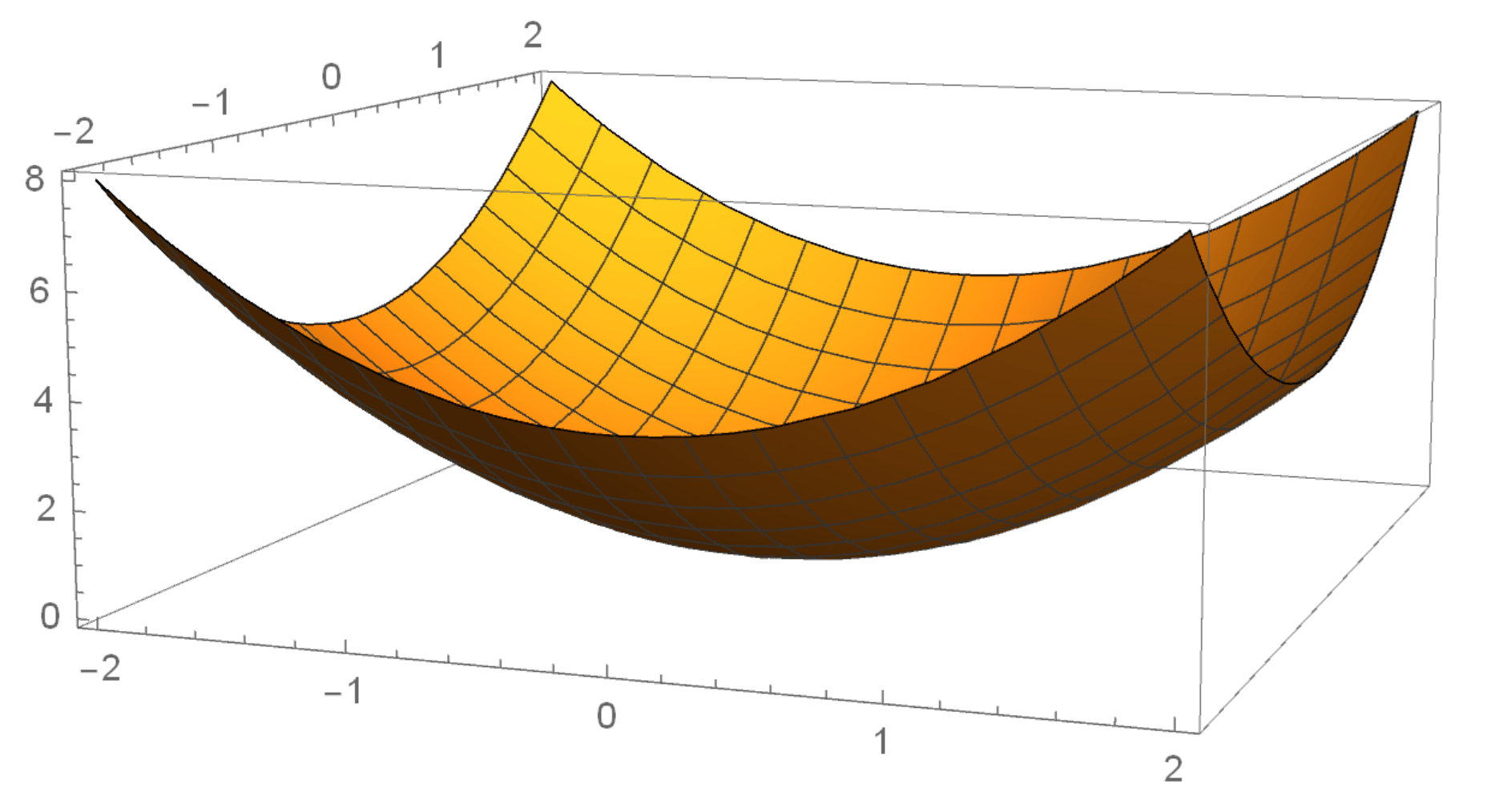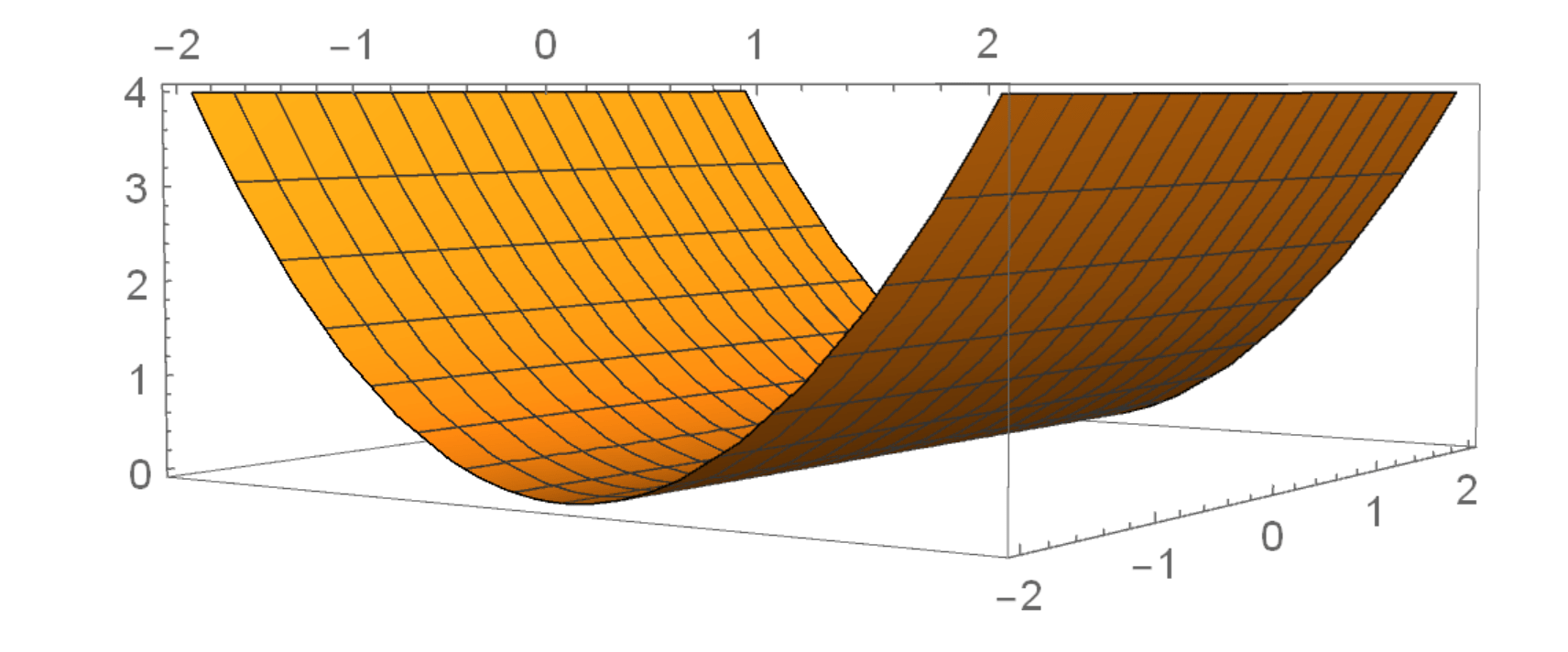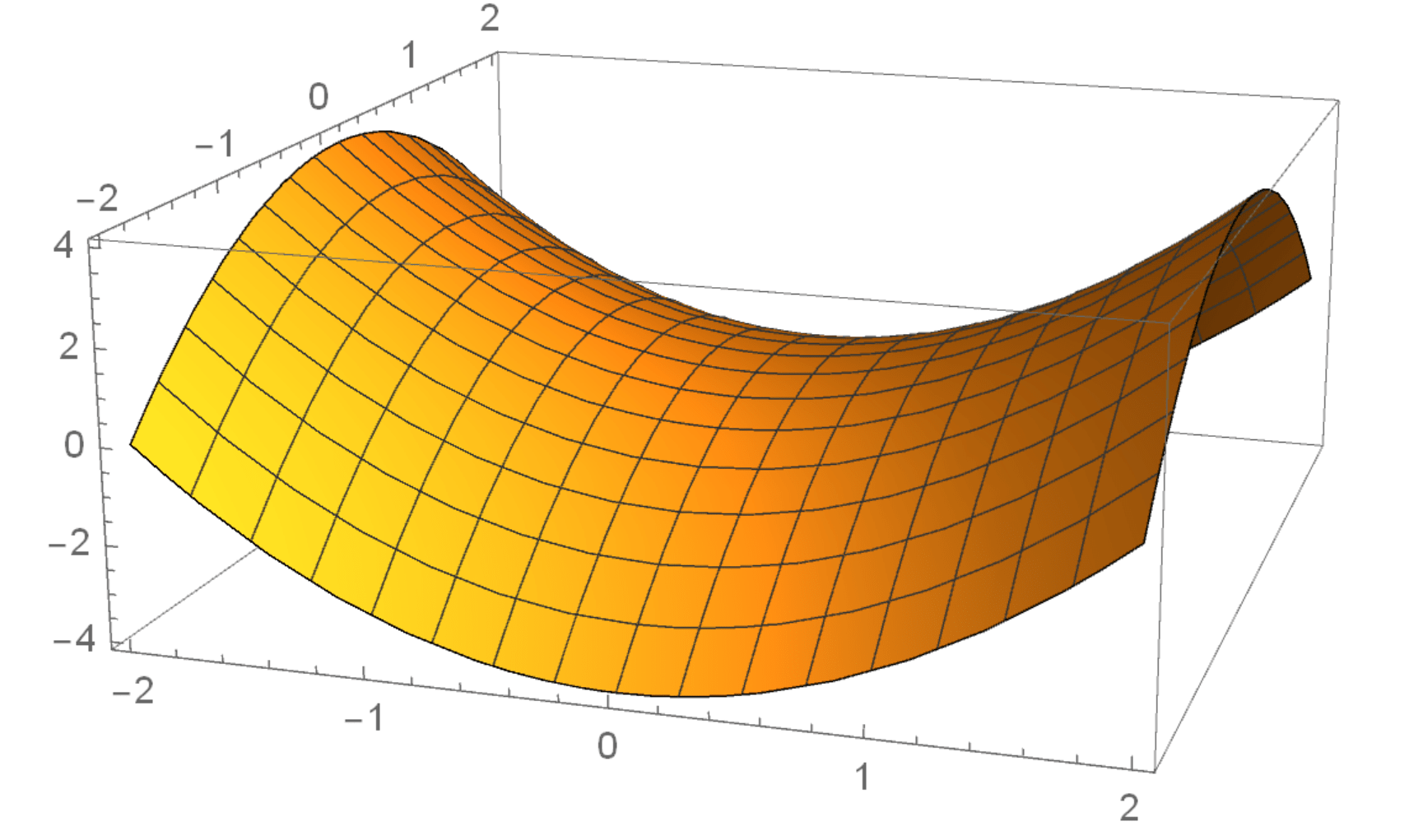15
Aug 18

## Law and order in the set of matrices

The law is to feel by touch every little fact. The order is discussed below.

### Why do complex numbers come right before this topic?

The analog of a conjugate number is the transpose$A^T.$ How can you tell this? Using Exercise 2 we see that$(cx)\cdot {y}=x\cdot (\bar{c}y).$ This looks similar to the identity$(Ax)\cdot y=x\cdot(A^Ty)$ (see Exercise 4). Therefore the mapping$c\rightarrow \bar{c}$ is similar to$A\rightarrow A^{T}.$

Once you know this, it's easy to come up with a couple of ideas.

Idea 1. From the characterization of real numbers (see Equation (4)) we see that matrices that satisfy$A=A^T$ (symmetric matrices) correspond to real numbers and should be better in some way than asymmetric ones.

Idea 2. From Equation (3) we see that the matrix$A^TA$ should be symmetric and non-negative.

### What is a non-negative matrix?

The set of real numbers is ordered in the sense that for any two real numbers$a,b$ we can say that either$a\geq b$ or$a is true. The most important property that we used in my class is this: if$a\geq b$ and$c>0,$ then$ac\geq bc$ (the sign of an inequality is preserved if the inequality is multiplied by a positive number). Since any two numbers can be compared like that, it is a complete order.

One way in which symmetric matrices are better than more general ones is that for symmetric matrices one can define order. The limitation caused by dimensionality is that this order is not complete (some symmetric matrices are not comparable).

Exercise 1. For the matrix$A=\left(\begin{array}{cc}a_{11}&a_{12}\\a_{12}&a_{22}\end{array}\right)$ and vector$x\in R^2$ find the expression$Q_A(x)=x^TAx.$ What is the value of this expression at$x=0?$

Solution$Q_A(x)=x_1^2+2a_{12}x_1x_2+a_{22}x_2^2$ and$Q_A(0)=0.$

Definition 1. The function$Q_A(x)=x^TAx$ is called a quadratic form of the matrix$A.$ Here$A$ is symmetric of size$n\times n$ and$x\in R^n.$

Discussion. 1) The facts that$A$ is in the subscript and the argument is$x$ mean that$A$ is fixed and$x$ is changing.

2) While the argument is a vector, the value of this function is a real number:$Q_A$ acts from$R^n$ to$R.$

3)$Q_A(x)$ does not contain constant or linear terms (of type$c$ and$ax_i).$ It contains only quadratic terms (write$x_1x_2=x_1^1x_2^1$ to see that the total power is 2), that's why it is called a quadratic form and not a quadratic function.

Definition 2. We say that$A$ is positive if$Q_A(x)>0$ for all nonzero$x\in R^n$ and non-negative if$Q_A(x)\geq 0$ for all$x\in R^n.$ (Most sources say positive definite instead of just positive and non-negative definite instead of just non-negative. I prefer a shorter terminology. If you don't understand why in the definition of positivity we require nonzero$x,$ go back to Exercise 1). As with numbers, for two symmetric matrices$A,B$ of the same size, we write$A>B$ or$A\geq B$ if$A-B$ is positive or non-negative, respectively. Continuing this idea, we can say that$A$ is negative if$-A$ is positive.

More on motivation. A legitimate definition of order$A\geq B$ would obtain if we compared the two matrices element-wise. Definition 2 is motivated by the fact that quadratic forms arise in the multivariate Taylor decomposition.

Sylvester's criterion is the only practical tool for determining positivity or non-negativity. However, in one case this is simple.

Exercise 2. Show that$A^TA$ is symmetric and non-negative.

Solution. The symmetry is straightforward and has been shown before. Non-negativity is not difficult either:$Q_{A^TA}(x)=x^TA^TAx=(Ax)^TAx=\|Ax\|^2\ge 0.$

### Geometry

The graph of a quadratic form in good cases is an elliptic paraboloid and has various other names in worse cases. Geometrically, the definition of the inequality$A\geq B$ means that the graph of$A$ is everywhere above the graph of$B$ (at the origin they always coincide). In particular,$A\geq 0$ means that the graph of$A$ is everywhere above the horizontal plane.

Examples. All examples are matrices of size$2\times 2$.Figure 1. Quadratic form of identity (elliptic paraboloid)

1) The identity matrix$I$ is positive because$Q_I(x)=x_1^2+x_2^2$, see Figure 1.Figure 2. Parabolic cylinder

2) The matrix$A=\left(\begin{array}{cc}1&0\\0&0\end{array}\right)$ is non-negative. Its quadratic form$Q_A(x)=x_1^2$ grows when$|x_1|$ grows and stays flat when$x_2$ changes and$x_1$ is fixed, see Figure 2.Figure 3. Hyperbolic paraboloid

3) The matrix$B=\left(\begin{array}{cc}1&0\\0&-1\end{array}\right)$ is not positive or non-negative or negative or non-positive. Its quadratic form$Q_B(x)$$=x_1^2-x_2^2$ is a parabola with branches looking upward when the second argument is fixed and a parabola with branches looking downward when the first argument is fixed, see Figure 3. When a surface behaves like that around some point, that point is called a saddle point.